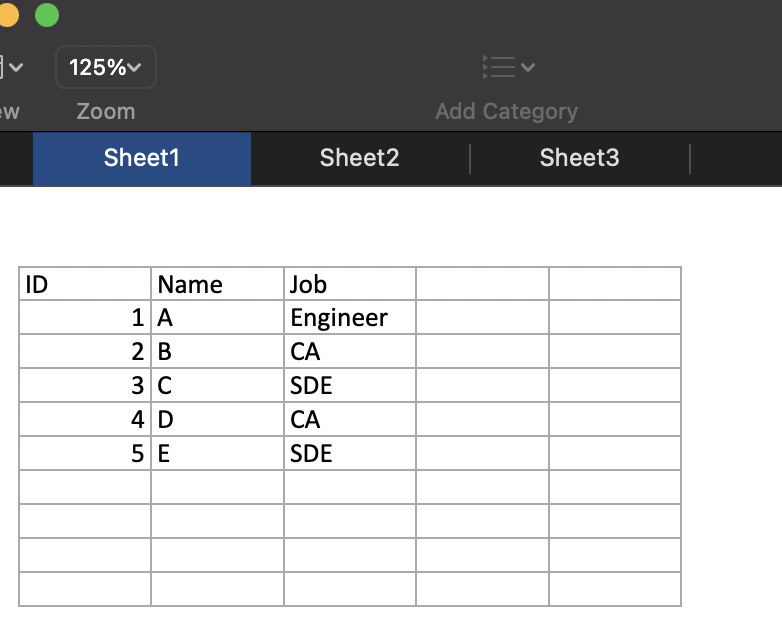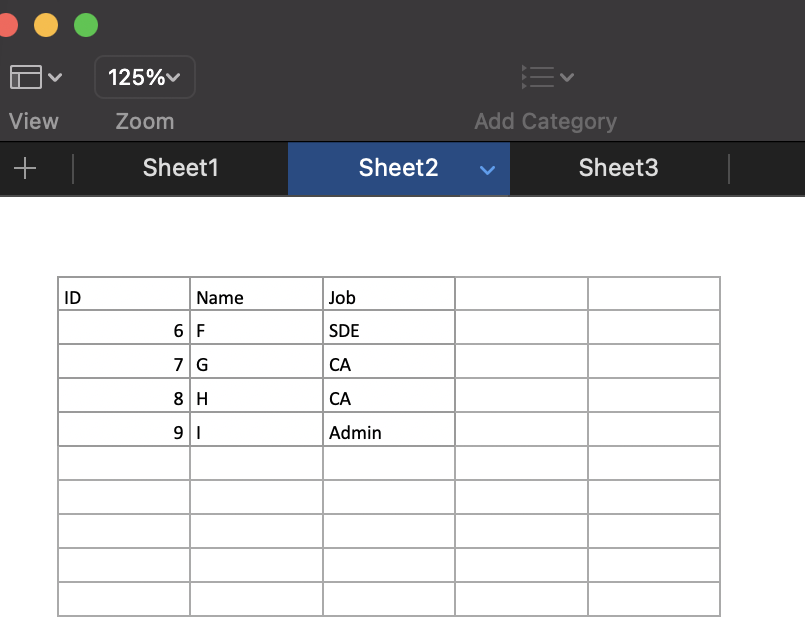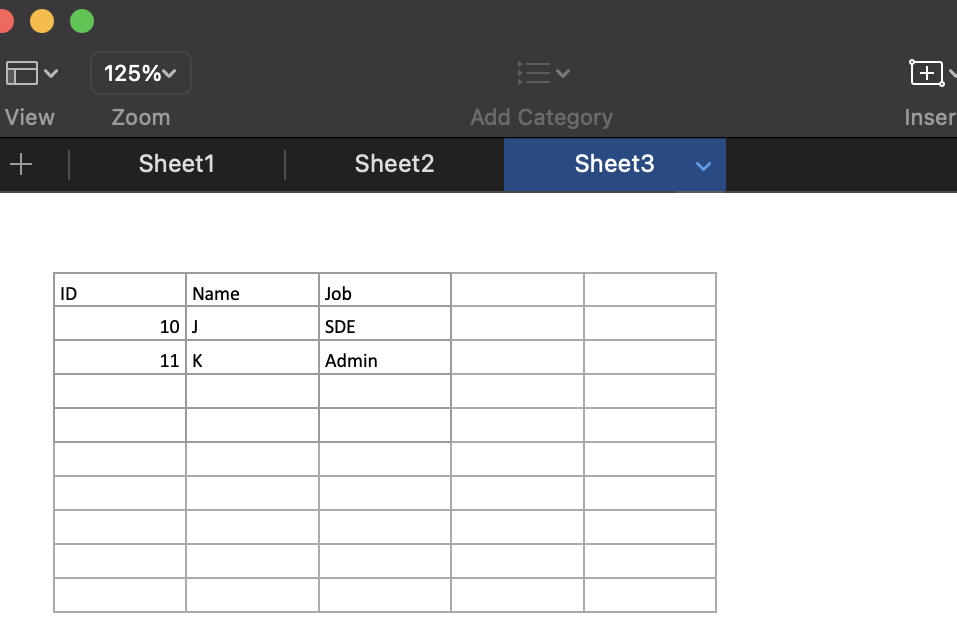GFG App
Open AppBrowser
Continue

# Combine Multiple Excel Worksheets into Single Dataframe in R

In this article, we will discuss how to combine multiple excel worksheets into a single dataframe in R Programming Language.

The below XLSX file “gfg.xlsx” has been used for all the different approaches.The inbuilt setwd() method is used to set the working directory in R.

The readxl package in R is used to import and read Excel workbooks in R, which can be used to easily work and modify the .xslsx sheets. It can be installed and loaded into the R working space using the following syntax  :

`install.packages("readxl")`

Initially, the excel_sheets() method is invoked to fetch all the worksheet names contained in the Excel workbook, with the specified file path.

`excel_sheets(path)`

The lapply() method in R is used to apply a function (either user-defined or pre-defined) to a set of components contained within an R list or dataframe. The lapply( ) method returns an object of the same length as that of the input object.

Syntax: lapply( obj , FUN)

Arguments:

obj – The object to apply the function on

FUN – The function to be applied  over different components of the object obj.

The FUN is the read_excel method of this package store, which is used to read the contents of the specified sheet name into a tibble, which is a tabular-like structure used to store data in fixed rows and columns. The lapply method applies the read_excel method over every sheet of the workbook.

Arguments:

path – The file path

sheet – The sheet name to read

The final tibble is produced by the inbuilt method bind_rows(), which takes as input the individual dataframes and assigns them a singular ID attribute key. The output of bind_rows() will contain a column if that column appears in any of the inputs. The output tibble contains the records contained in all the sheets , along with their respective data types. The column name “Sheet” is used to lead all the rows by forming a primary column.

## R

 `# installing the required libraries  ` `library``(readxl) ` `library``(tidyverse) ` ` `  `# specifying the path for file ` `path <- ``"/Users/mallikagupta/Desktop/"` ` `  `# set the working directory  ` `setwd``(path) ` ` `  `# accessing all the sheets  ` `sheet = ``excel_sheets``(``"Gfg.xlsx"``) ` ` `  `# applying sheet names to dataframe names ` `data_frame = ``lapply``(``setNames``(sheet, sheet),  ` `                    ``function``(x) ``read_excel``(``"Gfg.xlsx"``, sheet=x)) ` ` `  `# attaching all dataframes together ` `data_frame = ``bind_rows``(data_frame, .id=``"Sheet"``) ` ` `  `# printing data of all sheets ` `print ``(data_frame)`

Output

```# A tibble: 11 x 4
Sheet     ID Name  Job
<chr>  <dbl> <chr> <chr>
1 Sheet1     1 A     Engineer
2 Sheet1     2 B     CA
3 Sheet1     3 C     SDE
4 Sheet1     4 D     CA
5 Sheet1     5 E     SDE
6 Sheet2     6 F     SDE
7 Sheet2     7 G     CA
8 Sheet2     8 H     CA
10 Sheet3    10 J     SDE
11 Sheet3    11 K     Admin   ```

Method 2: Using rio package

The rio package is used to stimulate quick and easy data import and export operations to be performed in R. Rio makes deductions about the file format itself which can be used to read files easily.

`install.packages("rio")`

The import() and export() methods in R determine the data structure of the specified file extension. The method import_list() imports a list of dataframes from a multi-object file, for instance, an Excel workbook or an R zipped file.

Syntax: import_list(file, rbind = FALSE)

Arguments :

file – The file name of the Excel workbook to access

rbind – Indicator of whether to combine or not the dataframes into a single dataframe

## R

 `# specifying the path name ` `path <- ``"/Users/mallikagupta/Desktop/Gfg.xlsx"` ` `  `# importing the required library ` `library``(rio) ` ` `  `# reading data from all sheets ` `data <- ``import_list``(path , rbind=``TRUE``) ` ` `  `# print data ` `print ``(data)`

Output

```  ID Name      Job  _file
1   1    A Engineer     1
2   2    B       CA     1
3   3    C      SDE     1
4   4    D       CA     1
5   5    E      SDE     1
6   6    F      SDE     2
7   7    G       CA     2
8   8    H       CA     2